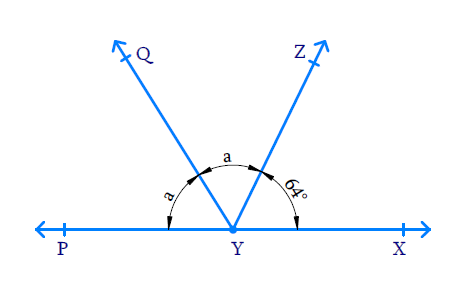# Ex.6.1 Q6 Lines and Angles Solution - NCERT Maths Class 9

Go back to  'Ex.6.1'

## Question

It is given that $$\angle XYZ = 64^ {\circ}$$ and $$XY$$ is produced to point $$P.$$ Draw a figure from the given information. If ray $$YQ$$ bisects $$\angle ZYP,$$ find $$\angle XYQ$$ and reflex $$\angle QYP.$$

Video Solution
Lines And Angles
Ex 6.1 | Question 6

## Text Solution

What is known?

$$\angle XYZ = 64^ {\circ}$$ and Ray $$YQ$$ bisects $$\angle PYZ.$$

What is unknown?

$$\angle XYQ = ?$$ and Reflex $$\angle QYP = ?$$

Reasoning:

When a ray intersects a line sum of adjacent angles formed is $$180^ {\circ}.$$

Steps:

With the given information in the question, we can come up with this diagram.Ray $$YQ$$ bisects $$\angle ZYP$$ and let $$\angle ZYQ = \angle QYP = a.$$

We can see from figure that $$PX$$ a line and $$YZ$$ is a ray intersecting at point $$Y$$ and the sum of adjacent angles so formed is $$180^ {\circ}.$$

Hence $$\angle ZYP + \angle ZYX = 180 ^ { \circ }$$

\begin{align}\angle ZYQ + \angle QYP + &\angle ZYX = 180 ^ { \circ } \\ a + a + 64 ^ {\circ } & = 180 ^ { \circ } \\ 2 a + 64 ^ {\circ } & = 180 ^ { \circ } \\ 2 a & = 180 ^ { \circ }-64 ^ {\circ } \\&= 116 ^ { \circ } \\ a & = \frac { 116 } { 2 } \\ & = 58 ^ { \circ } \end{align}

Then $$\angle XYQ = \angle XYZ + \angle ZYQ$$

\begin{align}\angle XYQ &= a + 64 ^ {\circ } \\ &= 58 ^ { \circ } + 64 ^ {\circ} \\ &= 122 ^ { 0 } \end{align}

As,

\begin{align}\angle QYP &= a, \text {Reflex}\angle QYP \\&= (360 ^ { \circ } - a) \end{align}

\begin{align}&= (360 ^ { \circ } - 58 ^ { \circ }) \\ &= 302 ^ { \circ } \end{align}

Reflex,$$\angle QYP = 302 ^ { \circ }$$

Video Solution
Lines And Angles
Ex 6.1 | Question 6

Learn from the best math teachers and top your exams

• Live one on one classroom and doubt clearing
• Practice worksheets in and after class for conceptual clarity
• Personalized curriculum to keep up with school